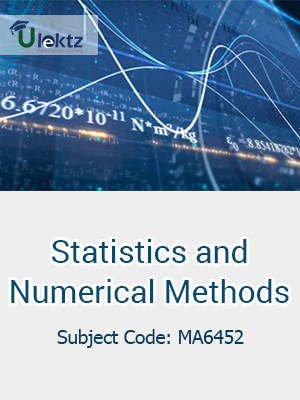uLektz apps

•My WalletMy Order
•My Profile
•My Connections
•My Books
•My Videos
•My Tests
•My Calender
•My Messages
•My Shopping Cart
•My Orders
•Account Settings
•Help

uLektz apps

Book DetailsStatistics And Numerical Methods

Course Code:MA6452

Author:Ulektz

University:

Regulation:2013

Categories:Mechanical

Format :ePUB3 (DRM Protected)

Type :eBook

FREE

Description :Statistics And Numerical Methods of MA6452 covers the latest syllabus prescribed by Anna University, Tamil Nadu for regulation 2013. Author: Ulektz, Published by uLektz Learning Solutions Private Limited.

Note : No printed book. Only ebook. Access eBook using uLektz apps for Android, iOS and Windows Desktop PC.

Topics
UNIT I TESTING OF HYPOTHESIS

1.1 Large sample test based on Normal distribution for single mean and difference of means

1.2 Tests based on t, χ2 and F distributions for testing means and variances

1.3 Contingency table (Test for Independency)

1.4 Goodness of fit

UNIT II DESIGN OF EXPERIMENTS

2.1 One way and two way classifications - Completely randomized design – Randomized block design –Latin square design

2.5 22 factorial designs

UNIT III SOLUTION OF EQUATIONS AND EIGENVALUE PROBLEMS

3.1 Newton Raphson method

3.2 Gauss elimination method- Pivoting

3.3 Gauss Jordan methods

3.4 Iterative methods of Gauss Jacobi and Gauss Seidel

3.5 Matrix inversion by Gauss Jordan method

3.6 Eigen values of a matrix by power method

UNIT IV INTERPOLATION, NUMERICAL DIFFERENTIATION AND NUMERICAL INTEGRATION

4.1 Lagrange’s and Newton’s divided difference interpolations

4.2 Newton’s forward and backward difference interpolation

4.3 Approximation of derivative using interpolation polynomials

4.4 Numerical single and double integration's using Trapezoidal and Simpson’s 1/3 rules.

UNIT V NUMERICAL SOLUTION OF ORDINARY DIFFERENTIAL EQUATIONS

5.1 Taylor’s series method

5.2 Euler’s method - Modified Euler’s method

5.3 Fourth order Runge-Kutta method for solving first order equations

5.4 Milne’s predictor corrector methods for solving first order equations

5.5 Finite difference methods for solving second order equations.

Related Books